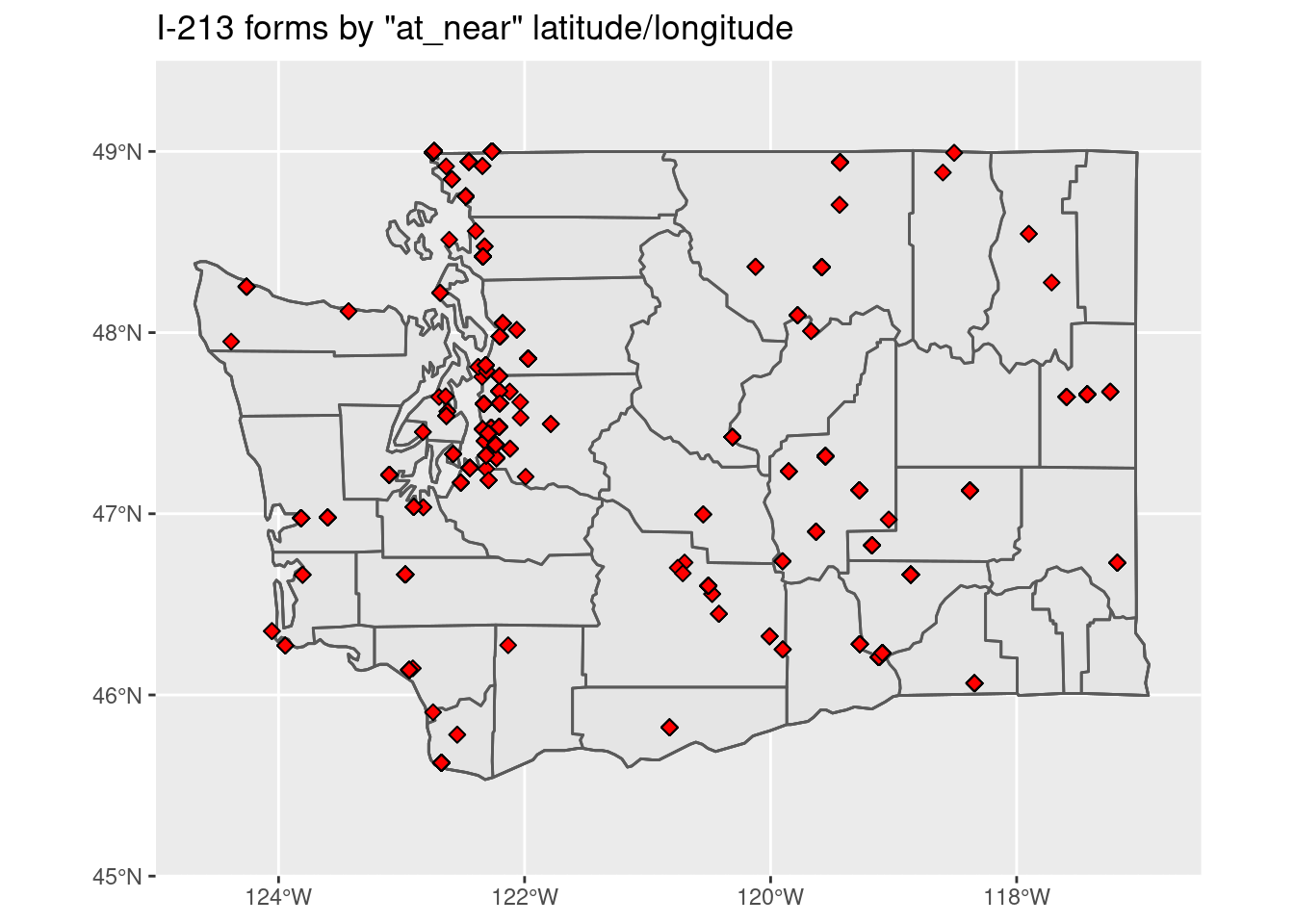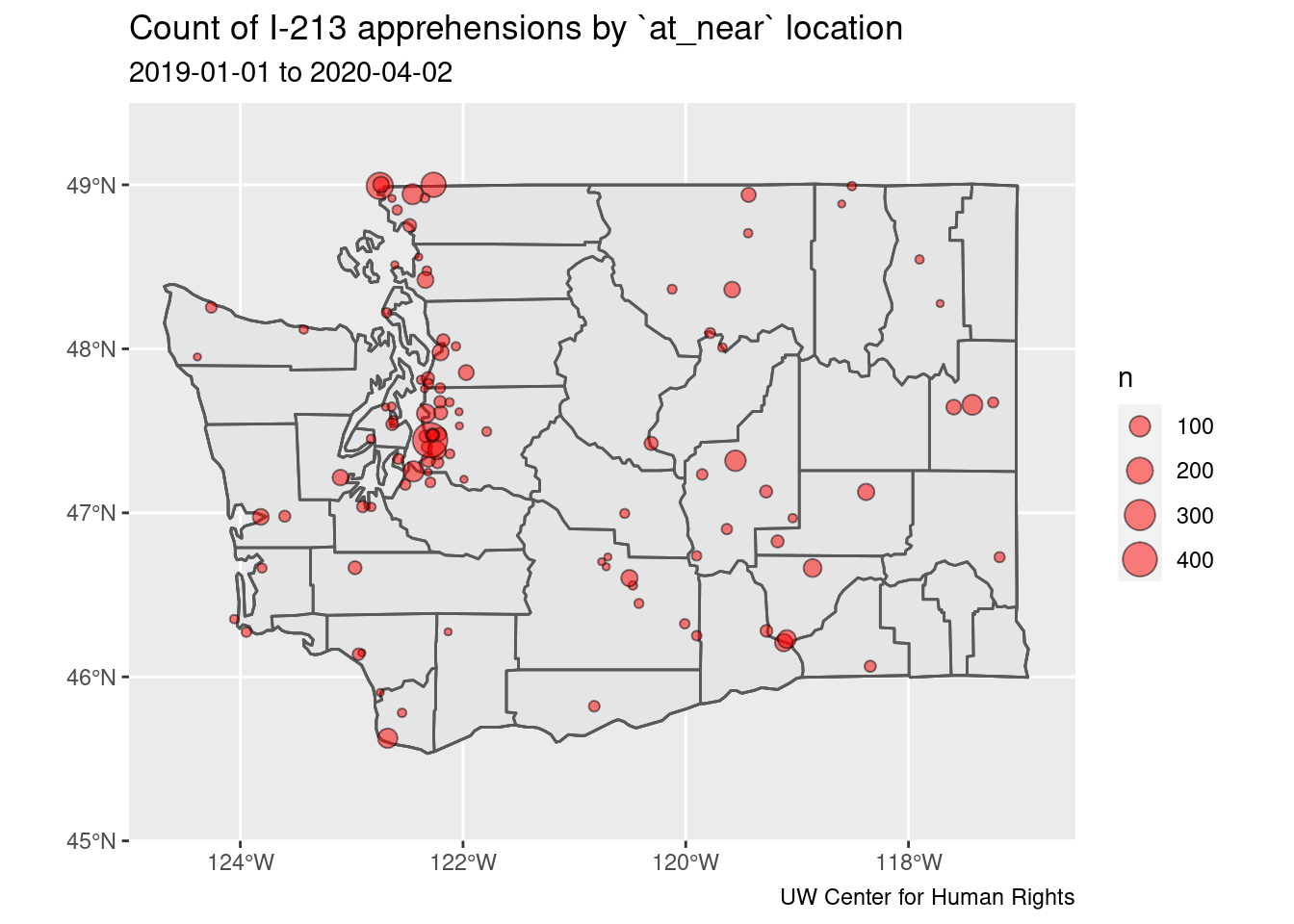# WA state I-213 apprehensions

This notebook performs preliminary descriptive analysis and mapping of ICE/CBP apprehensions in Washington state, based on a collection of I-213 forms obtained by the University of Washington Center for Human Rights (UWCHR) via a Freedom of Information Act (FOIA) lawsuit against the US Department of Homeland Security.

Data used in this analysis were scraped from PDF forms and cleaned in a separate private repository. I-213 narratives and other potentially sensitive fields have been excluded from analysis. Data scraping and cleaning code available for review upon request.

``````knitr::opts_chunk\$set(echo = TRUE)

pacman::p_load(tidyverse, lubridate, here, skimr, yaml, ggplot2, ggalt, ggsflabel,
sf, rnaturalearth, rnaturalearthdata, maps, tools, RColorBrewer
)

inputfile <- here::here('map', 'input', 'uw-chr-i213-public.csv.gz')

i213 <- read_delim(inputfile, delim = "|",
col_types = cols(
.default                     = col_character(),
source                       = col_factor(),
sex                          = col_factor(),
year                         = col_double(),
month                        = col_double(),
day                          = col_double(),
hour                         = col_double(),
minute                       = col_double(),
age                          = col_double(),
accompanied_juvenile_flag    = col_double(),
unaccompanied_juvenile_flag  = col_double(),
custody_redetermination_flag = col_double())
)

i213 <- i213 %>%
filter(source == 'ice',
state == 'WA',
year >= '2019')

priority_counties <- gsub(" County", "", priority_counties)
priority_counties <- tolower(priority_counties)

priority_plus <- append(priority_counties, 'whatcom')

i213\$date <- as.Date(i213\$date)

min_date <- min(i213\$date, na.rm = TRUE)
max_date <- max(i213\$date, na.rm = TRUE)``````

## Mapping I-213 apprehensions by `at_near` location

Most (but not all) I-213 forms include a field labeled “At/Near” that describes the location of the apprehension, usually as a “city, state” pair, but occasionally using other values such as the name of a jail or prison, or agency code for points of entry, such as “SEA” (SeaTac Airport) or “PHY” (Pacific Highway in Blaine, WA). Where “At/Near” values are ambiguous or missing, UWCHR has confirmed apprehension location based on I-213 narratives. After cleaning, locations are assigned latitude/longitude values using Google Maps API.

``````world <- ne_countries(scale = "medium", returnclass = "sf")

states <- st_as_sf(map("state", crs = 4326, plot = FALSE, fill = TRUE))

states <- cbind(states, st_coordinates(st_centroid(states)))
states\$ID <- toTitleCase(as.character(states\$ID))

wa <- states %>%
filter(ID == 'Washington')

counties <- st_as_sf(map("county", plot = FALSE, fill = TRUE))

counties <- counties %>%
separate(col = 'ID', into = c("state", "county"), sep = ",", remove = FALSE) %>%
filter(state == 'washington')

counties\$area <- as.numeric(st_area(counties))

sites <- i213 %>% select(lon, lat) %>%
na.omit()

sites <- st_as_sf(sites, coords = c("lon", "lat"),
crs = 4326, agr = "constant")

wa_coords <- coord_sf(xlim = c(-125, -116.5), ylim = c(45, 49.5), expand = FALSE, crs = 4326)

st_crs(wa) <- 4326
st_crs(wa\$geom) <- 4326
st_crs(sites) <- 4326
st_crs(sites\$geometry) <- 4326

ggplot(data = wa) +
geom_sf() +
geom_sf(data = wa, fill = NA) +
geom_sf(data = counties, fill = NA) +
geom_sf(data = sites, size = 2, shape = 23, fill = "red") +
wa_coords +
ggtitle('I-213 forms by "at_near" latitude/longitude')``````Note distribution of arrests throughout the state, with larger clusters along the northern border in Whatcom County and in SeaTac, locaiton of both the Seattle-Tacoma International Airport and SeaTac Federal Detention Center.

``````data_at_near <- i213 %>%
filter(year >= '2019') %>%
group_by(at_near, lon, lat) %>%
summarise(n = n()) %>%
na.omit()

data_at_near <- st_as_sf(data_at_near, coords = c("lon", "lat"),
crs = 4326, agr = "constant")

data_at_near\$rank <- rank(data_at_near\$n)

p1 <- ggplot(data = wa) +
geom_sf() +
geom_sf(data = wa, fill = NA) +
geom_sf(data = counties, fill = NA) +
geom_sf(data = data_at_near, aes(size=n), shape = 21, fill = "red", alpha=.5) +
wa_coords +
labs(x = NULL, y = NULL,
title = "Count of I-213 apprehensions by `at_near` location",
subtitle = paste(min_date, max_date, sep = ' to '),
caption = "UW Center for Human Rights")

p1````````````ggsave('wa-i213-count.png',
plot = p1,
device = 'png',
path = '../output',
scale = 1,
dpi = 300)

p2 <- ggplot(data = wa) +
geom_sf() +
geom_sf(data = wa, fill = NA) +
geom_sf(data = counties, fill = NA) +
geom_sf(data = data_at_ne``````